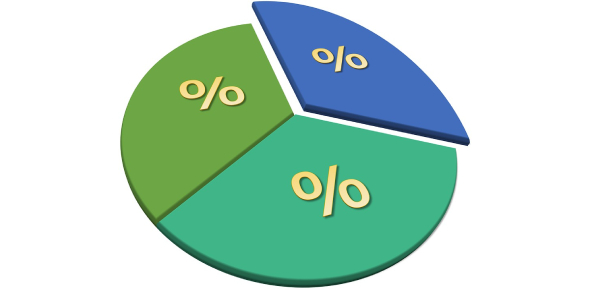# Percentage Test: Ultimate Math Quiz!

10 Questions | Total Attempts: 779Settings.

• 1.
What is the decimal equivalent of 33%?
• A.

3.3

• B.

33.0

• C.

0.33

• D.

0.033

• E.

None of the above

• 2.
What is the percentage equivalent of 0.5?
• A.

5%

• B.

0.5%

• C.

50%

• D.

500%

• E.

None of the above

• 3.
What is the decimal equivalent of 100%?
• A.

1.00

• B.

10.0

• C.

100.0

• D.

None of the above

• 4.
What is the percent equivalent of .755?
• A.

7.55%

• B.

75.5%

• C.

755.0%

• D.

None of the above

• 5.
How do you change a decimal to a percentage?
• A.

Just place a % sign after the numbers

• B.

Multiply the decimal by 10.

• C.

Multiply the decimal by 50.

• D.

Multiply the decimal by 100.

• 6.
How would you change a percentage from a percent to a decimal?
• A.

Place a decimal point between any of the numbers.

• B.

Place a decimal point before the last number.

• C.

Divide the percent by 100.

• D.

Divide the percent by 10.

• 7.
Which is the greater number 15% or _________
• A.

0.125

• B.

0.150

• C.

0.113

• D.

0.20

• E.

None of the above

• 8.
Which percent is equal to 0.25?
• A.

250%

• B.

2.5%

• C.

2500%

• D.

None of the above

• 9.
Four students are buying a large pizza with 12 slices. John paid for 25% of the pizza. How many slices should he get?
• A.

1 slice

• B.

2 slices

• C.

3 slices

• D.

4 slices

• E.

None, because I don't want to share!

• 10.
Your parents ask you and your two brothers to split the chores equally between all of you. There are 6 chores. What is the approximate percentage of chores that your two brothers will have to do?
• A.

25%

• B.

40%

• C.

33%

• D.

50%

• E.

I will have to do all of the chores because my brothers are lazy.

Related TopicsBack to top